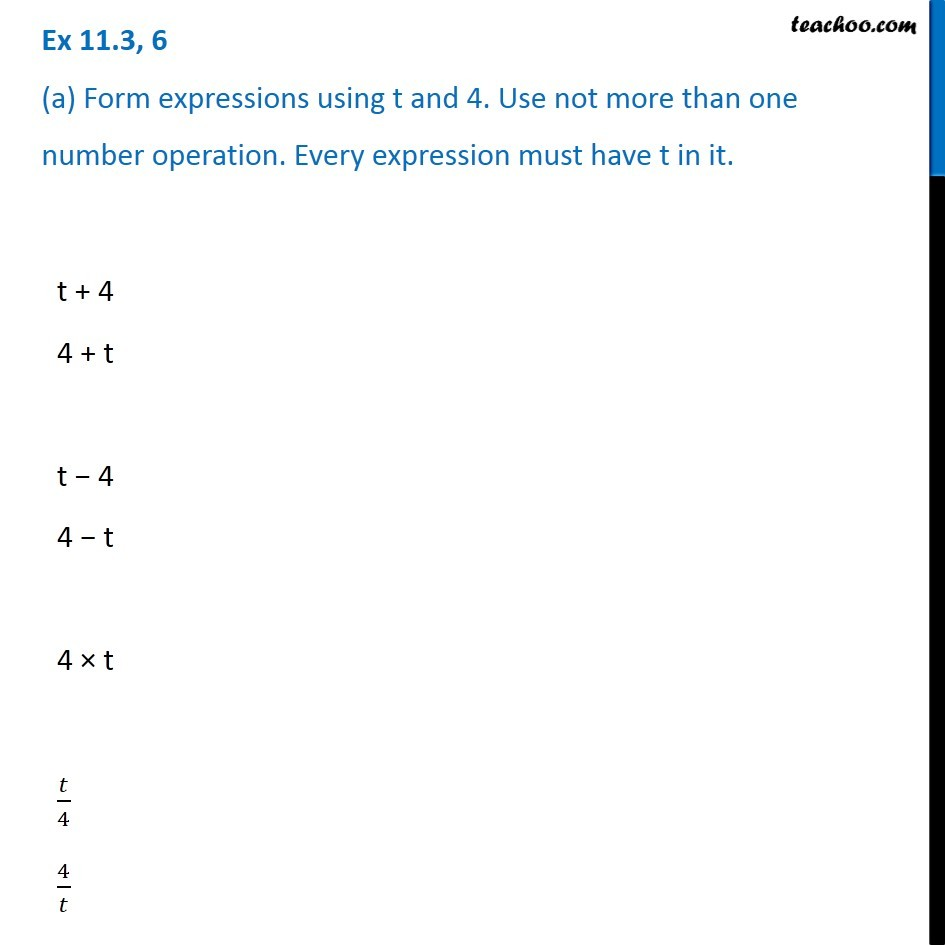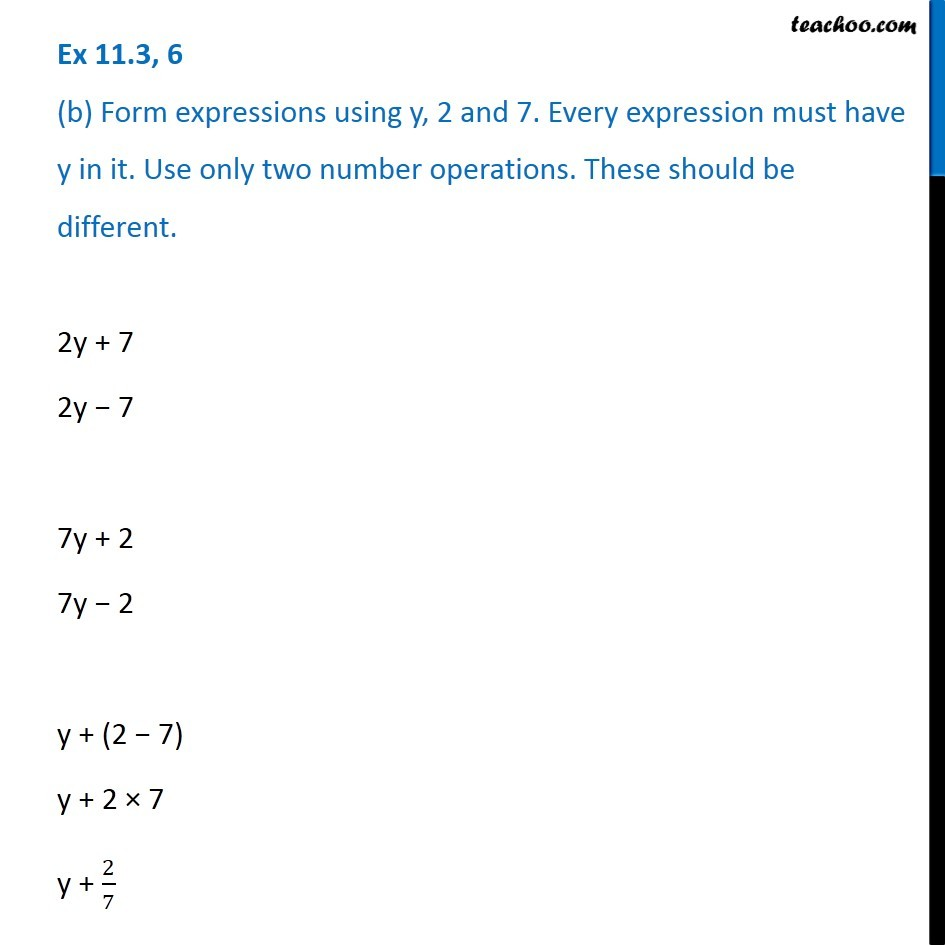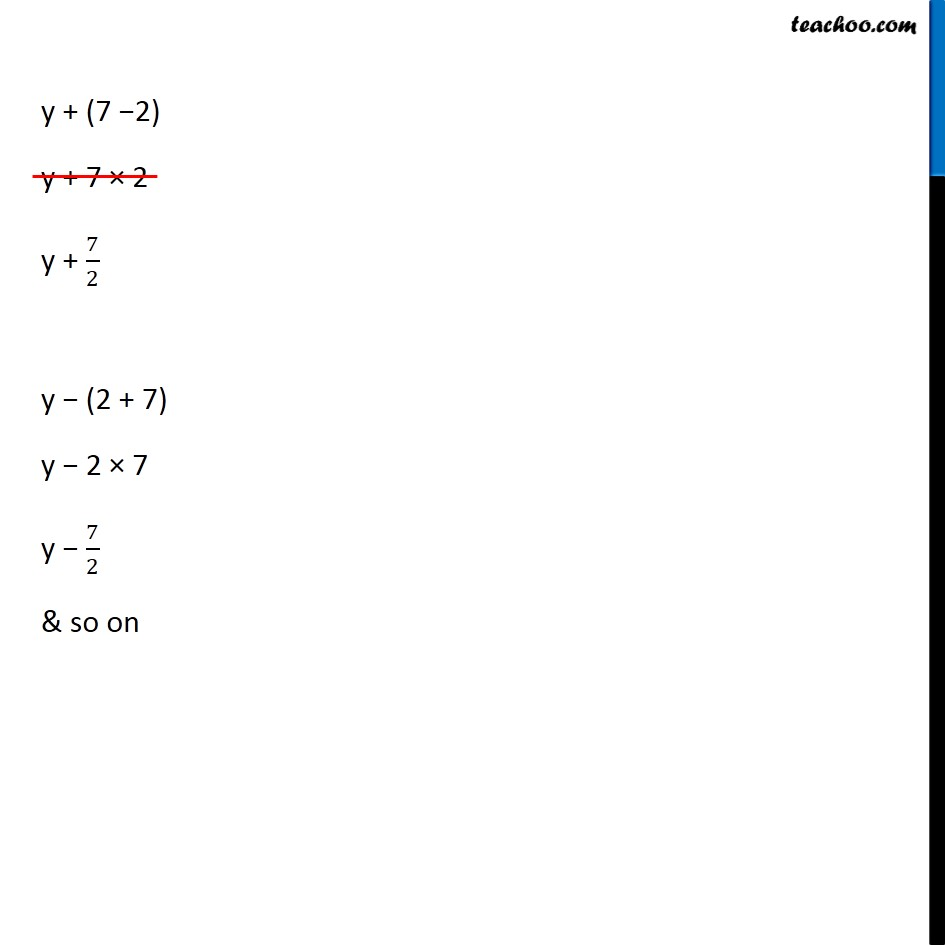1. Chapter 11 Class 6 Algebra
2. Concept wise
3. Expression with variables

Transcript

Ex 11.3, 6 (a) Form expressions using t and 4. Use not more than one number operation. Every expression must have t in it. t + 4 4 + t t − 4 4 − t 4 × t 𝑡/4 4/𝑡 Ex 11.3, 6 (b) Form expressions using y, 2 and 7. Every expression must have y in it. Use only two number operations. These should be different.2y + 7 2y − 7 7y + 2 7y − 2 y + (2 − 7) y + 2 × 7 y + 2/7 y + (7 −2) y + 7 × 2 y + 7/2 y − (2 + 7) y − 2 × 7 y − 7/2 & so on

Expression with variables

About the AuthorDavneet Singh
Davneet Singh is a graduate from Indian Institute of Technology, Kanpur. He has been teaching from the past 9 years. He provides courses for Maths and Science at Teachoo.